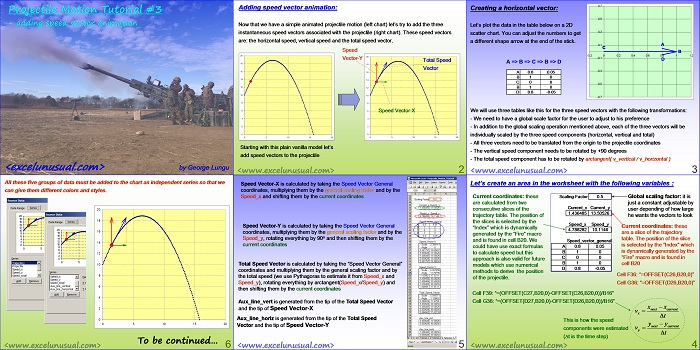# 2D Projectile Motion Tutorial #3

Now that we have a simple animated projectile motion (previous tutorial) let’s try to add on the chart the three instantaneous speed vectors associated with the projectile.

These speed vectors are: the horizontal speed, vertical speed and the total speed vector. The model works in all Excel versions but in 2007 it’s rather slow.[sociallocker][/sociallocker]

## Projectile Motion Tutorial #3 – adding speed vector animation

by George Lungu

Now that we have a simple animated projectile motion (left chart) let’s try to add the three
instantaneous speed vectors associated with the projectile (right chart). These speed vectors
are: the horizontal speed, vertical speed and the total speed vector.
Speed
Vector-Y
Total Speed

Starting with this plain vanilla model let’s
add speed vectors to the projectile
<www.excelunusual.com> 2

### Creating a horizontal vector:

Let’s plot the data in the table below on a 2D scatter chart. You can adjust the numbers to get
a different shape arrow at the end of the stick.

-0.2 0 0.2 0.4 0.6 0.8 1 1.2

A => B => C => B => D

We will use three tables like this for the three speed vectors with the following transformations:

– We need to have a global scale factor for the user to adjust to his preference

– In addition to the global scaling operation mentioned above, each of the three vectors will be
individually scaled by the three speed components (horizontal, vertical and total)

– All three vectors need to be translated from the origin to the projectile coordinates

– The vertical speed component needs to be rotated by +90 degrees

– The total speed component has to be rotated by arc-tangent( v_vertical / v_horizontal )

<www.excelunusual.com> 3

### Let’s create an area in the worksheet with the following variables :

Current coordinates:these are calculated from two consecutive slices of the trajectory table.
The position of the slices is selected by the “Index” which is dynamically generated by the “Fire” macro and is found in cell B20.
We could have use exact formulas to calculate speed but this approach is also valid for future models which use numerical methods to derive the position of the projectile.

Global scaling factor:it is just a constant adjustable by user depending of how large he wants the vectors to look
Current coordinates:these are a slice of the trajectory table.
The position of the slice is selected by the “Index” which is dynamically generated by the “Fire” macro and is found in cell B20

Cell F36: “=OFFSET(C26,B20,0)”
Cell G36: “=OFFSET(D26,B20,0)”
Cell F39: “=(OFFSET(C27,B20,0)-OFFSET(C26,B20,0))/B16”
Cell G36: “=(OFFSET(D27,B20,0)-OFFSET(D26,B20,0))/B16”
x * x
next, current
time
This is how the speed components were estimated
(t is the time step)

<www.excelunusual.com> 4

Speed Vector-X is calculated by taking the Speed Vector General
coordinates, multiplying them by the general scaling factor and by the
Speed_x and shifting them by the current coordinates.

Speed Vector-Y is calculated by taking the Speed Vector General
coordinates, multiplying them by the general scaling factor and by the
Speed_y, rotating everything by 90o and then shifting them by the
current coordinates.

Total Speed Vector is calculated by taking the “Speed Vector General”
coordinates and multiplying them by the general scaling factor and by
the total speed (we use Pythagoras to estimate it from Speed_x and
Speed_y), rotating everything by arctangent(Speed_x/Speed_y) and
then shifting them by the current coordinates.

Aux_line_vert is generated from the tip of the Total Speed Vector
and the tip of Speed Vector-X.

Aux_line_horiz is generated from the tip of the Total Speed
Vector and the tip of Speed Vector-Y.

<www.excelunusual.com> 5

All these five groups of data must be added to the chart as independent series so that we
can give them different colors and styles.

To be continued…

by George Lungu <www.excelunusual.com>